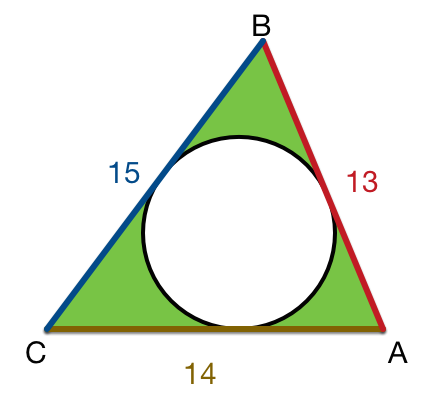# Circle With Triangle

Geometry Level 2In the figure above triangle $ABC$ with side-lengths $AC=14$, $AB=13$ and $BC=15.$ The incircle is drawn, which is tangential to all three sides. If the green shaded region is equal to $A$, find $\left\lfloor A\right\rfloor$.

×